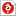검색어 입력폼

# 알고리즘 2장 연습문제

저작시기 2007.01 | 등록일 2007.04.20 | 최종수정일 2017.07.21한컴오피스 (hwp) | 3페이지 | 가격 10,000원

## 소개글

Fundamentals of Algorithms using C++ Pseudo code
2장 연습문제입니다.

2장 13번 문제.
2장 14번 문제.
2장 15번 문제.
2장 18번 문제.

## 본문내용

2장 13번 문제.
Write an algorithm that sorts a list of n items by dividing it into three sublists or almost n/3 items, sorting each sublist recurively and merging the three sorted sublists. Analyze your algorithm, and give the results using order notation.

2장 14번 문제.
Given the recurrence relateion

2장 15번 문제.
consider procedure solve(P,I,O) given below. This algorithm solves problem P by finding the output(solution) O corresponding to any input I.

2장 18번 문제.

When a divide-and-conquer algorithm divides an instance of size n of a problem into subinstances each of size n/c, the recurrence relation is typically given by

없음